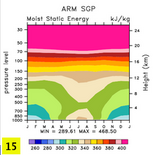f.e12.FAMIPC5.ne30_g16.amip_sst_cesm.001
and
f.e12.FAMIPC5.ne30_g16.amip.002

DIAG Set 15 - ARM Sites Annual Cycle Plots

Southern Great Plains (SGP)
T Temperature plot
Q Specific Humidity plot
CLOUD Cloud Fraction plot
MSE Moist Static Energy plot
TMQ Precipitable Water plot
FSDS Downwelling Shortwave Flux at Surface plot
FLDS Downwelling Longwave Flux at Surface plot
TGCLDLWP Total Grid-Box Cloud Liquid Water Path plot
CLDTOT Total Cloud plot
PRECT Precipitation plot
LHFLX Latent Heat Flux plot
SHFLX Sensible Heat Flux plot

T Temperature plot
Q Specific Humidity plot
CLOUD Cloud Fraction plot
MSE Moist Static Energy plot
TMQ Precipitable Water plot
FSDS Downwelling Shortwave Flux at Surface plot
FLDS Downwelling Longwave Flux at Surface plot
TGCLDLWP Total Grid-Box Cloud Liquid Water Path plot
CLDTOT Total Cloud plot

Tropical Western Pacific--Region 1 (TWP1)
T Temperature plot
Q Specific Humidity plot
CLOUD Cloud Fraction plot
MSE Moist Static Energy plot
TMQ Precipitable Water plot
FSDS Downwelling Shortwave Flux at Surface plot
FLDS Downwelling Longwave Flux at Surface plot
TGCLDLWP Total Grid-Box Cloud Liquid Water Path plot
CLDTOT Total Cloud plot

Tropical Western Pacific--Region 2 (TWP2)
T Temperature plot
Q Specific Humidity plot
CLOUD Cloud Fraction plot
MSE Moist Static Energy plot
TMQ Precipitable Water plot
FSDS Downwelling Shortwave Flux at Surface plot
FLDS Downwelling Longwave Flux at Surface plot
TGCLDLWP Total Grid-Box Cloud Liquid Water Path plot
CLDTOT Total Cloud plot

Tropical Western Pacific--Region 3 (TWP3)
T Temperature plot
Q Specific Humidity plot
CLOUD Cloud Fraction plot
MSE Moist Static Energy plot
TMQ Precipitable Water plot
FSDS Downwelling Shortwave Flux at Surface plot
FLDS Downwelling Longwave Flux at Surface plot
TGCLDLWP Total Grid-Box Cloud Liquid Water Path plot
CLDTOT Total Cloud plot

Surface Heat Budget of the Arctic Ocean (SHEBA)
FSDS Downwelling Shortwave Flux at Surface plot
FLDS Downwelling Longwave Flux at Surface plot